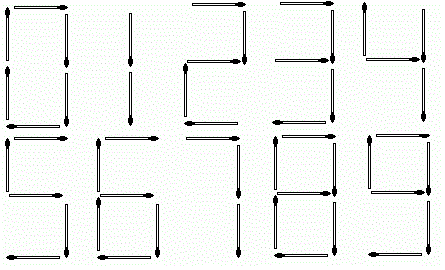304 North Cardinal St.
Dorchester Center, MA 02124

# Playing with Matches CodeChef Solution

## Problem – Playing with Matches CodeChef Solution

Chef’s son Chefu found some matches in the kitchen and he immediately starting playing with them.

The first thing Chefu wanted to do was to calculate the result of his homework — the sum of A and B, and write it using matches. Help Chefu and tell him the number of matches needed to write the result.

Digits are formed using matches in the following way:### Input

• The first line of the input contains a single integer T denoting the number of test cases. The description of T test cases follows.
• The first and only line of each test case contains two space-separated integers A and B.

### Output

For each test case, print a single line containing one integer — the number of matches needed to write the result (A+B).

• 1≤T≤1,000
• 1≤A,B≤10^6

### Sample 1:

``````Input:
3
123 234
10101 1010
4 4
Output:
13
10
7``````

### Explanation:

Example case 1: The result is 357. We need 5 matches to write the digit 3, 5 matches to write the digit 5 and 3 matches to write the digit 7.

Example case 2: The result is 11111. We need 2 matches to write each digit 1, which is 2⋅5=10 matches in total.

## Playing with Matches CodeChef Solution in C++17

``````#include <iostream>
using namespace std;

int main() {
int t;
cin>>t;
while(t--){
int a,b;
cin>>a>>b;
int s;
s=a+b;
int co=0;
int r;
while(s!=0)
{
r=s%10;
if(r==0||r==6||r==9)
{
co=co+6;
}
if(r==1)
{
co=co+2;
}
if(r==2||r==5||r==3)
{
co=co+5;
}
if(r==4)
{
co=co+4;
}
if(r==7)
{
co=co+3;
}
if(r==8)
{
co=co+7;
}
s=s/10;
}
cout<<co<<endl;
}
return 0;
}
``````

## Playing with Matches CodeChef Solution in Pyth 3

``````t=int(input())
for i in range(t):
l=[6,2,5,5,4,5,6,3,7,6]
a,b=map(int,input().split())
d=a+b
s=0
for i in str(d):
if(i=='0'):
s=s+l
elif(i=='1'):
s=s+l
elif(i=='2'):
s=s+l
elif(i=='3'):
s=s+l
elif(i=='4'):
s=s+l
elif(i=='5'):
s=s+l
elif(i=='6'):
s=s+l
elif(i=='7'):
s=s+l
elif(i=='8'):
s=s+l
else:
s=s+l
print(s)

``````

## Playing with Matches CodeChef Solution in Java

``````/* package codechef; // don't place package name! */

import java.util.*;
import java.lang.*;
import java.io.*;

/* Name of the class has to be "Main" only if the class is public. */
class Codechef
{
public static void main (String[] args) throws java.lang.Exception
{
Scanner sc = new Scanner(System.in);
int t= sc.nextInt();
while(t-->0){
int a = sc.nextInt();
int b = sc.nextInt();
int sum = a+b;
String s = String.valueOf(sum);
int m = 0;
for(int i=0;i<s.length();i++){
if(s.charAt(i)=='1'){
m = m+2;
}
else if(s.charAt(i)=='0'){
m = m+6;

}
else if(s.charAt(i)=='2'){
m= m+5;
}
else if( s.charAt(i)=='3'){
m = m+5;
}
else if(s.charAt(i)=='4'){
m = m+4;
}
else if(s.charAt(i) =='5'){
m=m+5;
}
else if(s.charAt(i) =='6'){
m = m+6;
}
else if(s.charAt(i)=='7'){
m = m+3;
}
else if(s.charAt(i)=='8'){
m = m+7;
}
else if(s.charAt(i)=='9'){
m = m+6;
}
else{
m=m;
}
}
System.out.println(m);
}
}
}
``````
##### Playing with Matches CodeChef Solution Review:

In our experience, we suggest you solve this Playing with Matches CodeChef Solution and gain some new skills from Professionals completely free and we assure you will be worth it.

If you are stuck anywhere between any coding problem, just visit Queslers to get the Playing with Matches CodeChef Solution

Find on CodeChef

##### Conclusion:

I hope this Playing with Matches CodeChef Solution would be useful for you to learn something new from this problem. If it helped you then don’t forget to bookmark our site for more Coding Solutions.

This Problem is intended for audiences of all experiences who are interested in learning about Data Science in a business context; there are no prerequisites.

Keep Learning!

More Coding Solutions >>

LeetCode Solutions

Hacker Rank Solutions

CodeChef Solutions